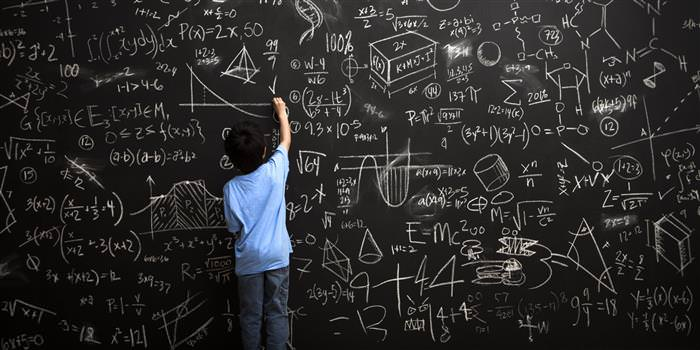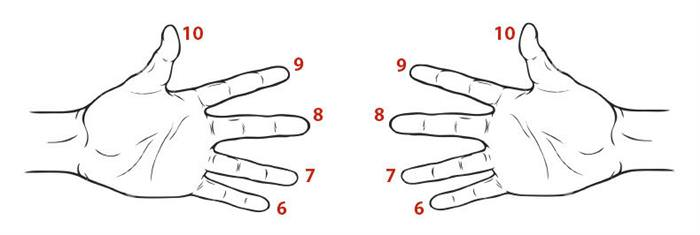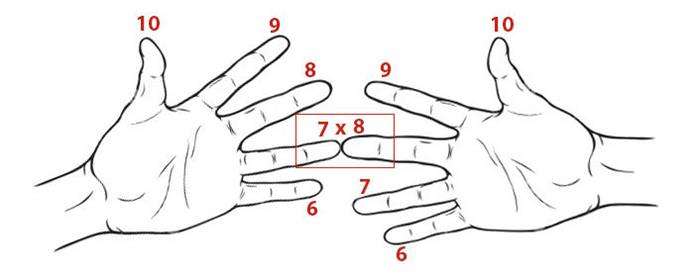# 7 Quick & Easy Math Tips They Should Teach at School

A+ A-
It’s been a while since we attended school, and age hasn’t been kind to our memory either. As time passes, most of us lose much of our mathematical abilities, leading us to rely more and more on calculators. If you want to improve your math skills, and even be able to help your kids, learn how to use these quick and easy methods.1. Calculate multiplications of 6,7,8,9 by using your fingers
Did you ever memorize the classic multiplication table? While calculating multiples of 1-5 are usually easy, when we go to 6 and higher, many of us start to stutter. This easy method is not taught in most schools, but it’s very quick and intuitive:
• Assign numbers to every finger, the pinky being number 6 and the thumb being number 10.• If you wish to calculate 7x8 (pictured below), touch the assigned fingers.• The number of fingers below, including the touching fingers, is the tens. (Pictured below, 5 fingers = 5 tens or 50)• Now, multiply the remaining fingers to find the units (in the example, 3 fingers multiplied by 2 fingers = 6).
• Add the units to the tens, and you have the result – 56.2. Quickly calculate multiples of 9
To calculate the multiples of nine, you just need to remember that the tens increase and the units decrease, and the sum of the total always equals nine.3. Calculating multiplications of eleven with 2-digit numerals
• This calculation takes two simple steps:
• In the first step, split the digits, where one becomes the hundreds, the other becomes the units.
• In the second step, sum the digits and the result becomes the tens.4. Quickly calculate fractions
Even though fractions are not something most of us deal with on a daily basis, this method is very useful, especially for school-age children.
• Turn the multiplication into a “butterfly” by drawing diagonal ellipses, then multiplying the digits in each ellipsis. (In the example below, 3x5 and 4x2)
• Write the results down in the appropriate “feeler”.• Multiply the denominators and write the answer under the butterfly. (In the example, 4x5=20)
• You now have the common denominator (20), and by adding the two numbers on the top of the butterfly, you have the numerator. (In the  example, 15+8=23)
• You can now simplify the fraction from 23/20 to 1+ 3/20.
• This method also works when you want to subtract fractions, but to do this, you will need to subtract the top numbers instead of adding them. (See example, 15-8=7)5. Multiplying double-digit numbers
Multiplication can become harder when the numbers get bigger, but it doesn’t have to be. This method will help you get over this, and you’ll find that the larger the number – the easier it gets:
• Subtract 100 from each number.
• Add the results, and then subtract it from 100. The result will become the thousands and hundreds digits.
• Now multiply the two first results and you’ll get the tens and units.6. Multiplying double and triple-digit numbers
Another method to multiply double-digit numbers is the “line method”, which also works on multiplying triple-digit numbers. It is vital that you remember to maintain a left-to-right writing direction, even when drawing the lines:
• Begin by drawing diagonal lines to represent each digit. The other number will be drawn in a crisscross manner shown in the example below.• Split the drawing into three sections. Each of the three areas represents the hundreds, tens, and units.• Count each cross-point in each section and write them down one after the other. (Left=1, middle=6, right=8)• To do the same for a triple-digit number, follow the same method, but notice that now you will have 4 sections instead of 3.7. For kids: How to write “greater than”
• Think of the sign as a hungry mouth that always wants to eat the bigger number!